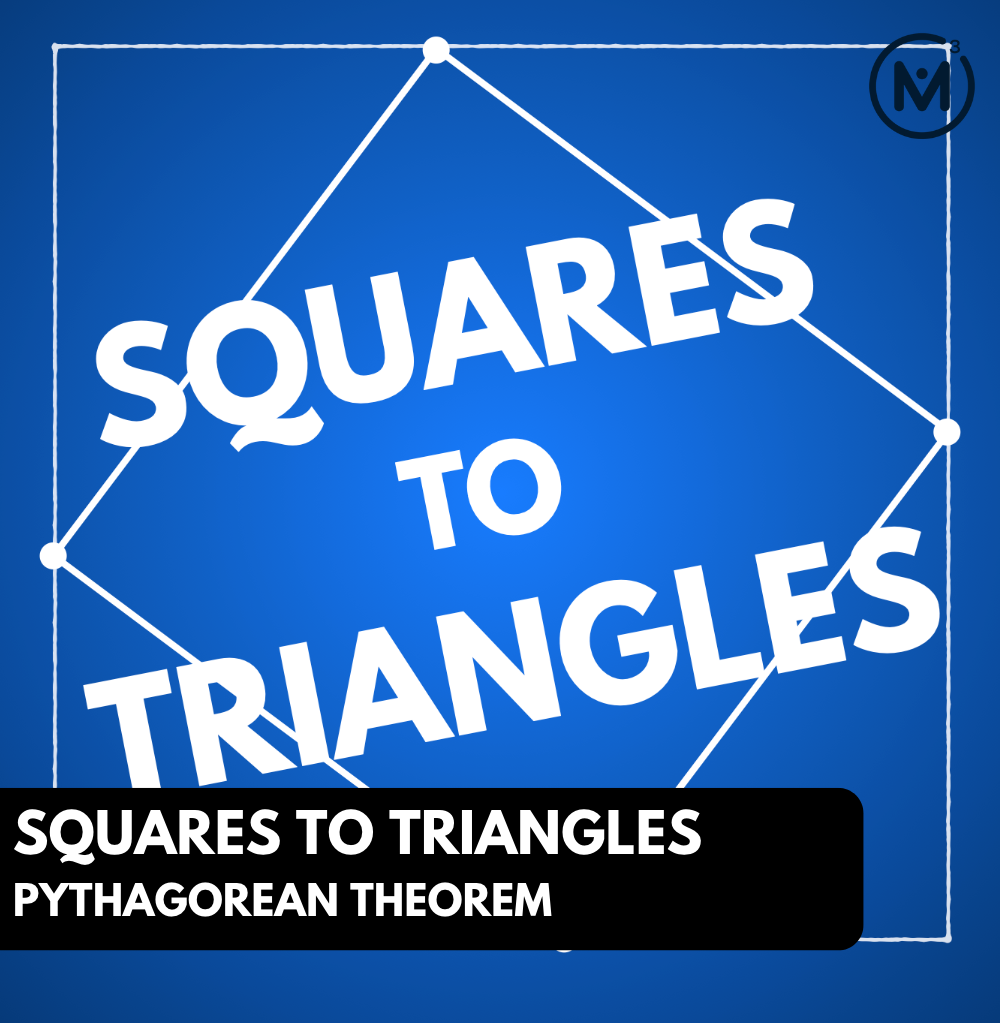## SQUARES TO TRIANGLES [DAY 3]

### PYTHAGOREAN THEOREM

Dive into our problem based unit where we explore the Pythagorean relationship through area of squares & triangles.

## Intentionality & Unit Overview### Access each lesson from this unit using the navigation links below

In this task students will practice determining various lengths in right triangles using the geometric model of the Pythagorean relationship as well as the symbolic model.

## Intentionality…

The purpose of the day 3 activities are to reinforce the ideas from day 1 and day 2. In this task students will use their understanding of areas of squares off the side lengths of right triangles to determine various lengths of the right triangle.

The math talk and purposeful practice serve to develop a deeper understanding of the Pythagorean relationship.

## Math Talk

Login/Join to access the entire Teacher Guide, downloadable slide decks and printable handouts for this lesson and all problem based units.

## While Students Are Practicing…

Login/Join to access the entire Teacher Guide, downloadable slide decks and printable handouts for this lesson and all problem based units.

## Purposeful Practice: The Pythagorean Theorem

Login/Join to access the entire Teacher Guide, downloadable slide decks and printable handouts for this lesson and all problem based units.

Login/Join to access the entire Teacher Guide, downloadable slide decks and printable handouts for this lesson and all problem based units.

## Educator Discussion Area

Login/Join to access the entire Teacher Guide, downloadable slide decks and printable handouts for this lesson and all problem based units.

## Explore Our 60+ Problem Based Units

This Make Math Moments Lesson was designed to spark curiosity for a multi-day unit of study with built in purposeful practice, number talks and extensions to elicit and emerge strategies and mathematical models.

Dig into our other units of study and view by concept continuum, grade or topic!